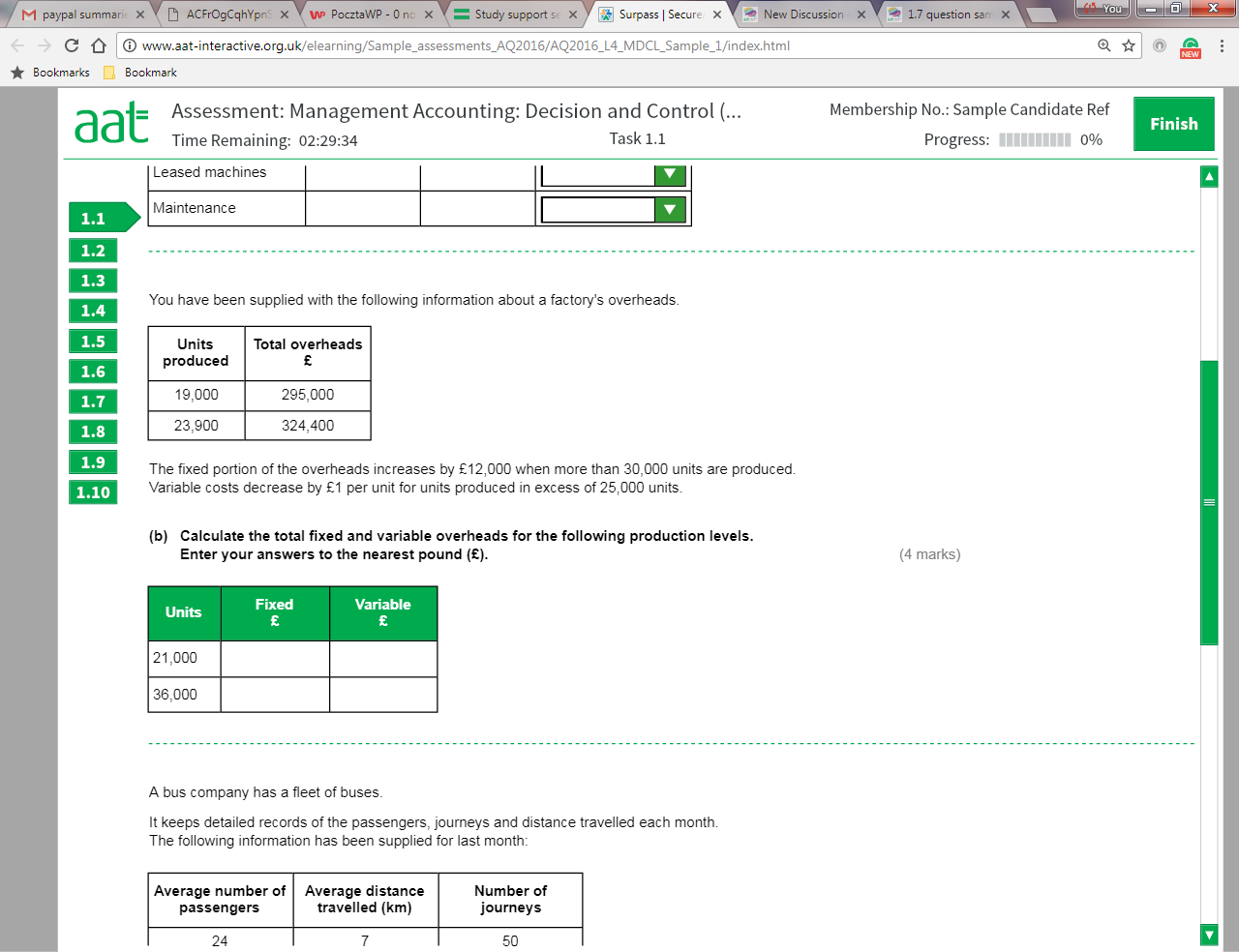Current updates regarding coronavirus (Covid-19) and the precautions AAT are taking will be continually updated on the below page.

We hope you are all safe and well and if you need us we will be here. 💚

# 1.1 B question sample assesment 1 Management Accounting: Decisions and Control

edited August 2017
How to calculate task 1.1 B fixed and variable overheads for the following productions level ? Any help?• Complete a high-low to get the variable, plug it back in for the fixed then take into account the amendments underneath the table.

23900u - £324400
19000u - £295000
4900u - £29400
1u - £6 variable
fixed costs: £181k

@ 21000u FC £181k VC £126k
@ 36000u FC £193k (£181k + £12k) VC £205k (25000 @ £6, 11000 @ £5)
• Thank you very much.
• How do you get the fixed costs of £181,000?

• Registered, MAAT, AATQB, AAT Licensed Accountant Posts: 116
If each unit has a variable cost of £6 then 23900 units will have variable costs of £143,400 Take this cost away from the full cost for 23900 units (£324,400 - £143,400) will give you £181,000 for the fixed costs. The same works for the 19000 units (19000 x 6 = £114,000). The full cost for 19000 units is £295,000 less the variable costs £114,000 = £181,000.

Hope that helps.
• Hi,

Please can someone explain this to me i've tried reading the other comments but it just doesn't make sense.

Thanks
Sophie
• Hi Sophie,

we have to produce data for 21000 and 36000 units and the condition that is given affects only the 36000 units, so finding the variable and fixed cost for 21000 will be straight forward by using the high low method on the data already given for 19000 and 23900 units, the calculation is as follows:
23900-19000=4900units
324400-295000=£29400
29400/4900 =£6 VARIABLE COST PER UNIT
Therefore 19000units x £6=£114000 variable cost
To find fixed cost we will use the formula total cost-variable cost=fixed cost, or 295000-114000=181000 fixed cost
We will use the same data for the 36000 units, but we will need to take into account the conditions given
We are told that anything above 30000 units the fixed cost will increase by £12000, therefore for the 36000 units the fixed cost must be 1810006+12000=193000
For the variable cost anything above 25000 units will cost £1 less, so:
25000units x £6=150000
11000 units x £5 =55000
Total 36000 units £205000
AAT Level 2. 2018 Distinction
AAT Level 3. 2018 Distinction
AAT Level 4. 2019 FSLC 100% MABU 93% MADC 82% PDSY 80%
• dumutroba said:

Hi Sophie,

we have to produce data for 21000 and 36000 units and the condition that is given affects only the 36000 units, so finding the variable and fixed cost for 21000 will be straight forward by using the high low method on the data already given for 19000 and 23900 units, the calculation is as follows:
23900-19000=4900units
324400-295000=£29400
29400/4900 =£6 VARIABLE COST PER UNIT
Therefore 19000units x £6=£114000 variable cost
To find fixed cost we will use the formula total cost-variable cost=fixed cost, or 295000-114000=181000 fixed cost
We will use the same data for the 36000 units, but we will need to take into account the conditions given
We are told that anything above 30000 units the fixed cost will increase by £12000, therefore for the 36000 units the fixed cost must be 1810006+12000=193000
For the variable cost anything above 25000 units will cost £1 less, so:
25000units x £6=150000
11000 units x £5 =55000
Total 36000 units £205000

Hi @dumutroba so helpful as always thanks so much!